Structural devices

This free course is available to start right now. Review the full course description and key learning outcomes and create an account and enrol if you want a free statement of participation.

Free course

# 6.2 Natural frequency of free oscillations

Most of us have a fairly accurate understanding of what is meant by resonance – it's what causes a bell to continue to make a sound long after it has been struck. Yet this is just one example of resonance, a phenomenon that occurs in nature in a surprisingly large number of places.

It is all to do with the reversible transfer of energy from one form to another in a system. The common feature associated with mechanical systems that are able to store energy by oscillating is that they have a moving mass and a restoring force. The energy in the system continually swaps back and forth, from kinetic energy in the form of the movement of the mass, to potential energy in the form of a displacement in the presence of a restoring force acting on the mass. The restoring force works to bring the mass to the point where the potential energy is a minimum and the kinetic energy is a maximum.

In the case of the AFM cantilever, the mass is that of the cantilever itself and the restoring force is provided by the elasticity of the material from which the cantilever is made.

I'll start by analysing a simpler system: a mass attached to a fixed location by a spring, as shown in Figure 24. If the mass is displaced from its rest position so as to extend or compress the spring, the spring will provide a restoring force. This, according to Hooke's law, is proportional to the displacement of the mass and in a direction so as to move the mass back to its rest position. In order to see the mathematics that relates to this look at the simple harmonic oscillator (see Box 8).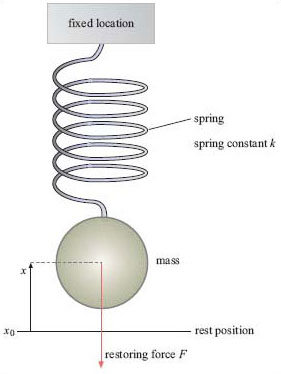Figure 24 A simple mass-spring system. The spring will always push or pull the mass towards its rest position, x0. If the mass is in motion it is likely that its momentum will carry it past its rest position

## Box 8 Simple harmonic oscillator

We can use the expression for Newton's second law of motion, F = ma (where F is the restoring force produced by the spring, m is the mass and a its acceleration), to analyse the mass-spring system and obtain an equation for how the displacement varies with time. After the mass has been displaced the restoring force F is given by the product of the spring constant, k, and the displacement, x (as shown in Figure 24):Force and displacement are both vectors so the negative sign is needed to show that the restoring force produced by the spring is in the opposite direction to the original displacement.

Acceleration is the rate of change of velocity, which in turn is the rate of change of displacement. Expressing the acceleration, a, in its differential form we have: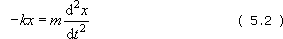Writing this in the standard form for second order differential equations gives: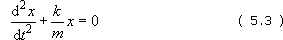Solutions to this type of equation take the general form: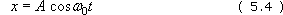This tells us that the displacement of the mass follows a sinusoidal pattern over time with natural angular frequency ω0 (in rad s−1) and amplitude of oscillation A (Figure 25). We can turn ω0 into a linear frequency by dividing by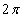(sinceradians are covered for every complete oscillation). This frequency, f0, is termed the natural frequency of oscillation.

If you are familiar with solving second-order differential equations, you will be aware that the general solution should be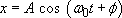. However, the φ term simply introduces a phase shift and we can ignore this if we choose to start the clock when t = 0.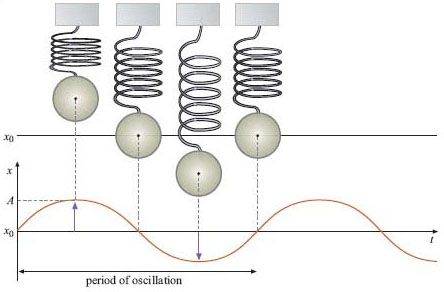Figure 25 This graph shows the position of the mass with respect to time. You can clearly see the maximum amplitude, A, and the period of oscillation

If you know how to differentiate expressions like A cos ω0t then you can easily verify this as a solution by differentiating it twice and inserting it into Equation (5.3). By doing this you can also verify that: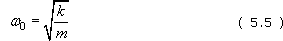If you have not met differentiation before then you can simply accept this solution. Either way, it is essential to note what the solution says about the behaviour of the oscillating system:

1. It goes as cos ωt, so the position, x, of the mass oscillates with time.

2. The natural frequency f0 is given by: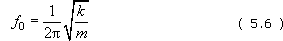(because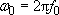). This depends on the spring constant and the mass, but not on the initial displacement. In particular you should note that a high spring constant leads to a high natural frequency.

3. The amplitude, A, does not vary with time.

Rewriting Equation (5.5) and substituting into Equation (5.3) gives: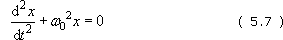which I will use later.

The analysis of the mass-spring system shows that the mass will oscillate with a frequency given by Equation (5.6) and a fixed amplitude, indefinitely. This is referred to as simple harmonic motion, often abbreviated to SHM. This is an approximation to what we observe in the real world for two reasons:

1. The amplitude will decay with time rather than remain constant.

The motion of a mass-spring system in the real world would suffer frictional losses (referred to as damping). These might be due to factors such as air resistance, internal friction in the material of the spring and/or energy lost to the spring's fixed location.

2. The mass-spring system will not oscillate unless something sets it in motion.

There are two distinct ways to set the system into motion:

• I can displace the mass then let go (as suggested before)

• I can drive the system continuously by shaking the spring at its ‘fixed’ location.

The amplitude A in the solution above is the initial displacement if there are no losses. I will discuss the case where the system is continuously driven later on; at this stage it is not represented in the analysis.

T356_1

### Take your learning further

Making the decision to study can be a big step, which is why you'll want a trusted University. The Open University has 50 years’ experience delivering flexible learning and 170,000 students are studying with us right now. Take a look at all Open University courses.

If you are new to university level study, find out more about the types of qualifications we offer, including our entry level Access courses and Certificates.

Not ready for University study then browse over 900 free courses on OpenLearn and sign up to our newsletter to hear about new free courses as they are released.

Every year, thousands of students decide to study with The Open University. With over 120 qualifications, we’ve got the right course for you.

Request an Open University prospectus